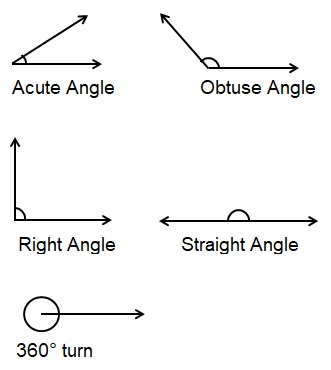# Angles

When two lines meet at a point, they form an angle at that point. An angle is actually the measurement of turn. It is measured in degrees e.g. 90° or 90 degree.

An angle gives an idea about the degree by which a line should be turned along its one end to overlap the other line.There are different types of angles

1) Acute Angle: If the angle is less than 90° then it is called an acute angle.

2) Obtuse Angle: If the angle is greater than 90° then it is called an obtuse angle.

3) Right Angle: If the angle is equal to 90° then it is called a right angle.

4) Straight angle: If the angle is equal to 180° then it is called a straight angle.Imp points:

• All internal angles of a triangle sum up to 180°.
• If we take a complete round turn we rotate by 360°.
• When a thing turns upside down it turns by 180°.